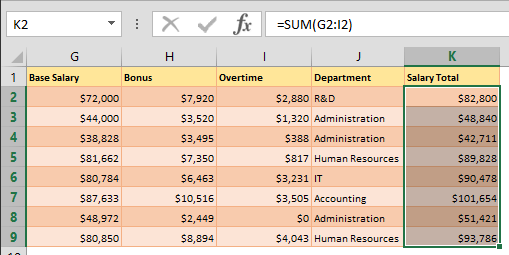Select PageHow to enable manual calculations if your Excel is running slow

As the number of formulas and data in your workbook increase, so will calculation times. If you’re at a point where you need to wait a while after every action, you should probably consider switching to manual calculations. In this article, we’re going to...How to copy a formula in Excel between workbooks without links using a text editor

You may have noticed that Excel generates links between workbooks, when you copy and paste a formula from one to the other. These links between worksheets call the original workbook for the formula you need in the present workbook. To get rid of them, you need to...How to copy a formula in Excel between workbooks without links using Find & Replace

You may have noticed that Excel generates links between workbooks, when you copy and paste a formula from one to the other. These links between worksheets call the original workbook for the formula you need in the present workbook. To get rid of them, you need to...